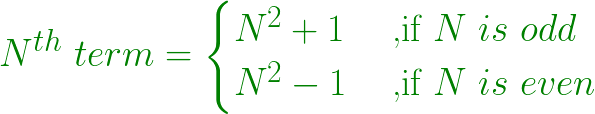# Find Nth term of the series 2, 3, 10, 15, 26….

• Last Updated : 20 Aug, 2022

Given a number N, the task is to find the Nth term in series 2, 3, 10, 15, 26….
Example:

```Input: N = 2
Output: 3
2nd term = (2*2)-1
= 3

Input: N = 5
Output: 26
5th term = (5*5)+1
= 26```

Approach:

• Nth number of the series is obtained by
1. Squaring the number itself.
2. If the number is odd, add 1 to the squared number. And, subtract 1 if the number is even
• Since the starting number of the series is 2
1st term = 2
2nd term = (2 * 2) – 1 = 3
3rd term = (3 * 3) + 1 = 10
4th term = (4 * 4) – 1 = 15
5th term = (5 * 5) + 1 = 26
and so on….

• In general, Nth number is obtained by formula:•

Below is the implementation of the above approach:

## C++

 `// C++ program to find Nth term``// of the series 2, 3, 10, 15, 26....` `#include ``using` `namespace` `std;` `// Function to find Nth term``int` `nthTerm(``int` `N)``{``    ``int` `nth = 0;` `    ``// Nth term``    ``if` `(N % 2 == 1)``        ``nth = (N * N) + 1;``    ``else``        ``nth = (N * N) - 1;` `    ``return` `nth;``}` `// Driver Method``int` `main()``{``    ``int` `N = 5;` `    ``cout << nthTerm(N) << endl;` `    ``return` `0;``}`

## Java

 `// Java program to find Nth term``// of the series 2, 3, 10, 15, 26....``class` `GFG``{` `// Function to find Nth term``static` `int` `nthTerm(``int` `N)``{``    ``int` `nth = ``0``;` `    ``// Nth term``    ``if` `(N % ``2` `== ``1``)``        ``nth = (N * N) + ``1``;``    ``else``        ``nth = (N * N) - ``1``;` `    ``return` `nth;``}` `// Driver code``public` `static` `void` `main(String[] args)``{``    ``int` `N = ``5``;` `    ``System.out.print(nthTerm(N) +``"\n"``);` `}``}` `// This code is contributed by Rajput-Ji`

## Python3

 `# Python3 program to find Nth term``# of the series 2, 3, 10, 15, 26....` `# Function to find Nth term``def` `nthTerm(N) :``    ``nth ``=` `0``;` `    ``# Nth term``    ``if` `(N ``%` `2` `=``=` `1``) :``        ``nth ``=` `(N ``*` `N) ``+` `1``;``    ``else` `:``        ``nth ``=` `(N ``*` `N) ``-` `1``;` `    ``return` `nth;` `# Driver Method``if` `__name__ ``=``=` `"__main__"` `:``    ``N ``=` `5``;``    ``print``(nthTerm(N)) ;` `# This code is contributed by AnkitRai01`

## C#

 `// C# program to find Nth term``// of the series 2, 3, 10, 15, 26....``using` `System;` `class` `GFG``{` `// Function to find Nth term``static` `int` `nthTerm(``int` `N)``{``    ``int` `nth = 0;` `    ``// Nth term``    ``if` `(N % 2 == 1)``        ``nth = (N * N) + 1;``    ``else``        ``nth = (N * N) - 1;` `    ``return` `nth;``}` `// Driver code``public` `static` `void` `Main(String[] args)``{``    ``int` `N = 5;` `    ``Console.Write(nthTerm(N) +``"\n"``);``}``}` `// This code is contributed by PrinciRaj1992`

## Javascript

 ``

Output:

`26`

Time Complexity: O(1) since no loop is used the algorithm takes up constant time to perform the operations

Auxiliary Space: O(1) since no extra array is used so the space taken by the algorithm is constant

My Personal Notes arrow_drop_up